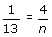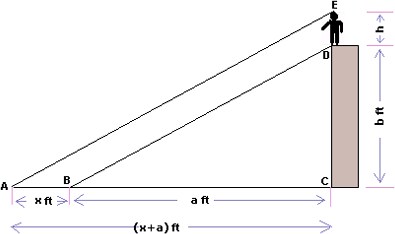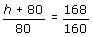## Definition Of Indirect Measurement

Indirect measurement is a technique that uses proportions to find a measurement when direct measurement is not possible.

### Example of Indirect Measurement

The length of a pole in a picture is given as 4 inches. The scale of the picture is given to be 1 inch : 13 inches. By setting up a proportion, the length n of the actual pole can be found.Solving the proportion, we get n = 52 inches.

### Solved Example on Indirect Measurement

#### Ques: A boy stands on the top of a building and the total length of shadows of the building and the boy is (x + a) ft and the length of the shadow of the boy alone is x ft. If the height of the building is b ft, then find the height of the boy. [Given x = 8, a = 160, b = 80.]##### Choices:

A. 4 ft
B. 3.5 ft
C. 3 ft
D. None of the aboveStep 5: 160(h + 80) = 168 × 80 [Write the cross products.]